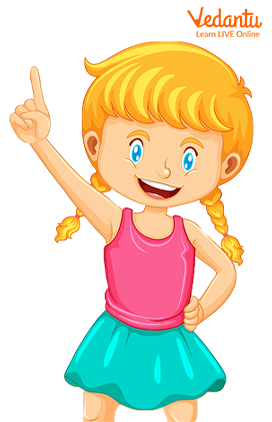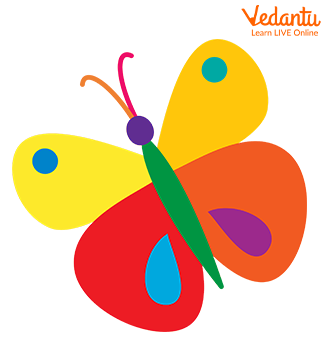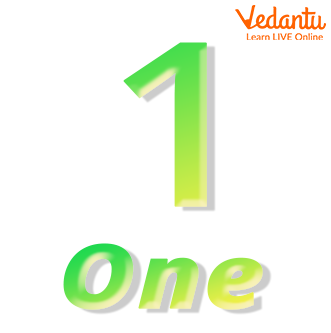Courses
Courses for Kids
Free study material
Free LIVE classes
More

# What is 1 and How to Write 1 in Words?## An Introduction to Number 1 for Kids

The concept of numbers is one of the preliminary concepts that is first taught to kids. Counting numbers is the very aspect of introducing kids to the subject of Mathematics. Only after mastering this, a child is introduced to mathematical operations like addition, subtraction, and so on. Let us look into the introduction to numbers for kids. In this article, we focus our discussion on answering what is 1? The article also mentions common rhymes that can be used to familiarise the number.

## What is 1?

The number 1 is the very first number in Mathematics. The counting of numbers begins with the number 1. One is a number in Mathematics that represents the quantity or the value of 1. While counting, the number 1 is succeeded by 2 which is then succeeded by 3 and so on. Before learning some interesting facts about the number 1, let us look into what a number is.

Number is defined as the mathematical term that is used to describe the quantity or value of objects. The number is generally used to quantify things.

## Counting the Number 1

We have learnt that numbers are primarily used for quantification, which includes counting things. Now let us look into examples where we can count the number of things and develop a better understanding of what is 1.

Example 1Image: Girl showing 1 finger

In the above-given image, the girl is holding up a single finger, which can also be said that the girl is showing 1 finger.

Example 2Image: A single or 1 butterfly

In the above-given image, there is a butterfly, if we were told to count the number of butterflies, we would answer that there is 1 butterfly.

Example 3Image: The image illustrates a balloon, a moon and a lion

In this image, there is one of everything; there is 1 balloon, 1 lion and 1 moon.

## How to Write Number 1 in Words?

As we have learnt what is 1, we can now answer the question of how to write 1 in words. 1 is written as “one” in words.Image: Number 1 in words

## Song to Learn the Number One

Planning an activity or memorising the facts through song or poem can help kids in developing a better understanding of the concept. Let us look into a song that can help kids to memorise number one.

One little funny clown,

Wears one shiny crown.

He dances around one town,

One skip, one hop and a turnaround.

## Conclusion

This was the complete discussion on what is 1. We have learnt about 1, the number; we have also learnt how to count to 1 and write 1 in words. As kids grow, they are introduced to various concepts. Teaching kids with the help of images and fun activities as mentioned in the article can help kids understand better. We hope that the article helps kids develop curiosity about numbers and Maths. If you enjoyed learning about the number 1 and would like to study the other numbers in a similar way, head to our website today!

Last updated date: 18th Sep 2023
Total views: 136.2k
Views today: 4.36k

## FAQs on What is 1 and How to Write 1 in Words?

1. Is one an odd number?

Yes, one is an odd number. An odd number is defined as the number that is not completely divisible by 2 since one is only divisible by one it is classified as an odd number.

2. How to teach kids to write number one?

Teaching kids how to write a number can be a fun experience for both parents and kids. Kids usually love colourful papers and images. Parents can print colourful papers that have dots that resemble the number. Teach kids to connect the dots, in this way, they can familiarise themselves with the structure of the number. Parents can also sing rhymes as mentioned in the article to help kids in better memorising and writing the number.